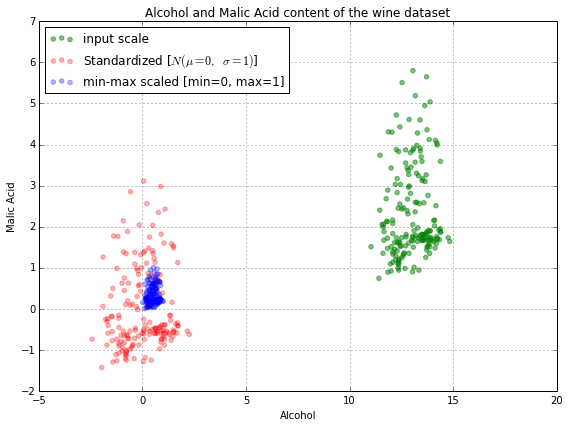2 views

I am using Linear regression to predict data. But, I am getting totally contrasting results when I Normalize (Vs) Standardize variables.

Normalization = x -xmin/ xmax – xmin

Zero Score

Standardization = x - xmean/ xstd

a) Also, when to Normalize (Vs) Standardize?

b) How Normalization affects Linear Regression?

c) Is it okay if I don't normalize all the attributes/labels in the linear regression?

Thanks, Santosh

by (33.1k points)

You simply need different hyperparameters for the two options to give similar results.

In most problem cases, standardization and normalization might simply help.

For clustering, standardization may be quiet crucial in order to compare similarities between features based on certain distance measures. Principal Component Analysis (PCA), but we prefer standardization over Min-Max scaling since we are interested in the components that maximize the variance.

There is a disadvantage of normalization over standardization is that it dissipates some information in the data, especially outliers.

For example:In the above image, scaling clusters is quite close together, that is unwanted in our case. It might cause algorithms such as gradient descent to take longer to converge on the same solution.

"Normalizing variables" is incorrect here. The correct term here is "normalizing/scaling the features".